• 基于matlab的GBP,IHT,IRLS压缩感知算法对比，同时拥有OMP，sp等算法的对比CS
• 实现了六种压缩感知算法，均为.m文件，可在matlab直接运行
• 1 压缩感知理论基础压缩感知(CS)技术处理信号的过程一般分为以下三个步骤：①信号的稀疏表示。CS要求信号具有稀疏性，若原始信号是稀疏的，则可直接用于后续操作；若原始信号具有稀疏性但不是稀疏的，则利用稀疏基将...


1 压缩感知理论基础压缩感知(CS)技术处理信号的过程一般分为以下三个步骤：①信号的稀疏表示。CS要求信号具有稀疏性，若原始信号是稀疏的，则可直接用于后续操作；若原始信号具有稀疏性但不是稀疏的，则利用稀疏基将其稀疏化；②观测矩阵(也称测量矩阵)。观测矩阵用于对原始信号进行压缩采样；③重构方法。重构是指从少量信息中恢复出大量数据，CS具有多种重构方法。图1是CS数学框架示意图。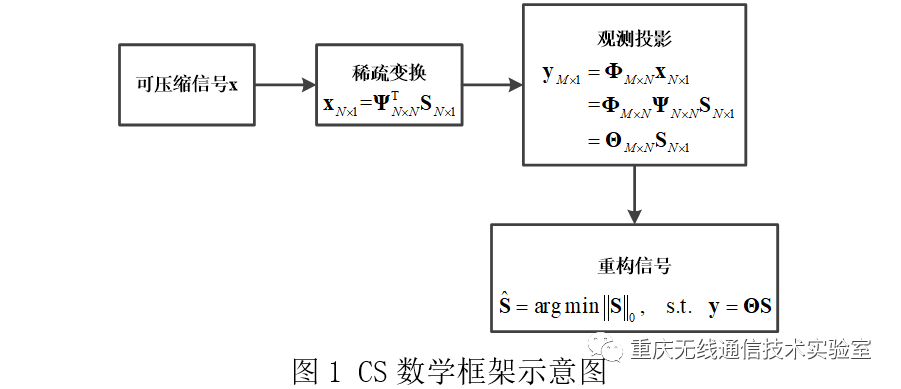2 OMP算法原理   正交匹配跟踪(OMP)算法是一种贪婪算法，其基本思想是在迭代过程中，每一次从感知矩阵A选择与残差余量最匹配的一个原子(即与残差最相关的列)，来构建稀疏信号，并计算出新的残差余量。由于每次只选择一个原子，因此被选择的原子与残差余量几乎正交，为保证不重复选择此原子，需从感知矩阵中删除这一列，然后再继续挑选与信号残差最匹配的一列，循环迭代，直至残差低于迭代停止条件。OMP方法的具体流程图如表1所示：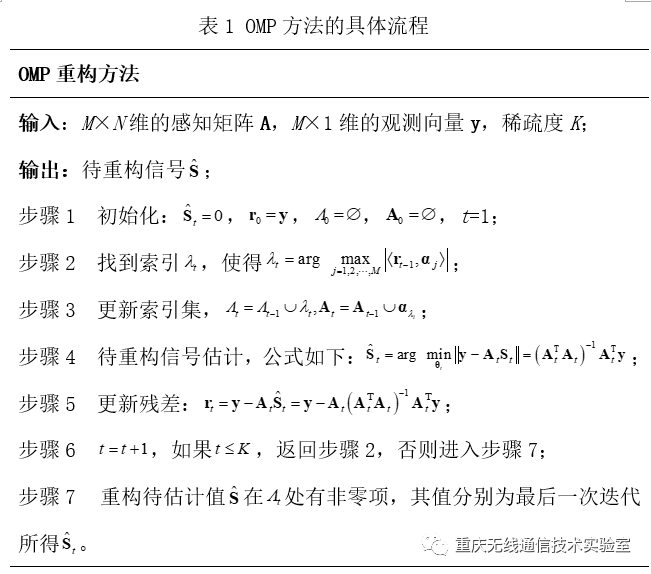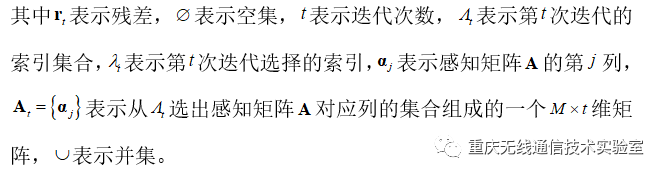3 OMP算法代码function [ theta ] = CS_OMP(y,A,t ) %   Detailed explanation goes here %   y = Phi * x %   x = Psi * theta %   y = Phi*Psi * theta %   令 A = Phi*Psi, 则y=A*theta %   现在已知y和A，求theta     [y_rows,y_columns] = size(y);  %矩阵y的大小为y_rows,y_columns    if y_rows        y = y';         %y shouldbe a column vector     end     [M,N] = size(A);       %传感矩阵A为M*N矩阵      theta = zeros(N,1);    %用来存储恢复的theta(一行N列向量，全0)      At = zeros(M,t);       %用来迭代过程中存储A被选择的列     Pos_theta = zeros(1,t); %用来迭代过程中存储A被选择的列序号      r_n = y;                %初始化残差(residual)为y    for ii=1:t             %迭代t次，t为输入参数         product = A'*r_n;  %传感矩阵A各列与残差的内积         [val,pos] = max(abs(product)); %找到最大内积绝对值，即与残差最相关的列        At(:,ii) = A(:,pos);      %存储这一列          Pos_theta(ii) = pos;      %存储这一列的序号         A(:,pos) = zeros(M,1);    %清零A的这一列，其实此行可以不要，因为它与残差正交         %y=At(:,1:ii)*theta，以下求theta的最小二乘解(Least Square)          theta_ls =(At(:,1:ii)'*At(:,1:ii))^(-1)*At(:,1:ii)'*y;%最小二乘解         %At(:,1:ii)*theta_ls是y在At(:,1:ii)列空间上的正交投影         r_n = y - At(:,1:ii)*theta_ls;%更新残差              end      theta(Pos_theta)=theta_ls;%恢复出的theta end 4 实验结果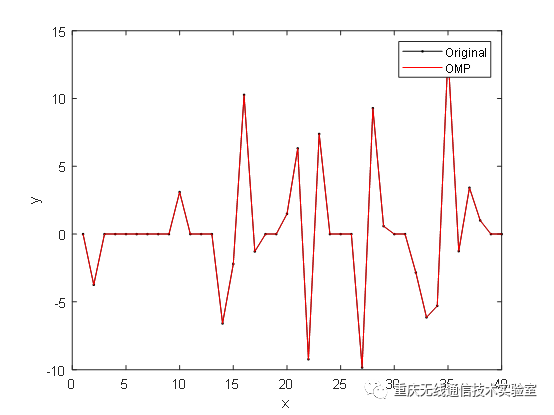实验结果表明，OMP算法可以对稀疏信号进行恢复并能达到较高的恢复精度。王帅，重庆大学无线通信技术实验室硕士研究生，主研方向为智能信号与信息处理。


展开全文• 机械振动信号分块自适应压缩感知算法.pdf,为提高振动信号分块压缩感知过程中的信号重构效果，提出了机械振动信号的自适应分块压缩感知算法。首先将信号分割，构造信号矩阵，并利用K SVD构造与信号矩阵相适应的冗余...
• 一篇关于贝叶斯压缩感知算法的原理以及应用的外文文献，比较详细
• 基于拉普拉斯分级先验模型的贝叶斯压缩感知算法,对图像进行合理分块,用不同比率对分块图像压缩,并在重构过程进行分级处理,进一步减少运算时间,最终使用相关向量机(RVM)实现了稀疏信号的最大后验概率估计。...
•研究论文
•研究论文
• MATLAB源码集锦-基于压缩感知算法的数据压缩与复原代码
• 成像专题 | 非均匀介质全息成像：基于压缩感知算法实现(OSA OE)Noise suppression for ballistic-photons based on compressive in-line holographic imaging through an inhomogeneous medium本期导读本期分享探讨...


成像专题 | 非均匀介质全息成像：基于压缩感知算法实现(OSA OE)Noise suppression for ballistic-photons based on compressive in-line holographic imaging through an inhomogeneous medium本期导读    本期分享探讨计算光学成像领域一个经典且实用的研究热点：散射介质成像。众所周知，全息成像过程会受到其他非均匀介质的影响，例如透过云雾成像、透过毛玻璃成像、透过浑浊液体水下成像及透过生物组织成像等。在多数实际应用层面，光路中的非均匀介质会在全息图中引入扰动噪声，进而严重影响成像质量。近日，来自清华大学和杜克大学的研究人员在光学领域顶级期刊Optics Express发表了研究工作，提出利用压缩感知(Compressive Sensing)算法实现透过散射介质高信噪比成像。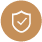技术路线    该论文提出了一种基于压缩采样的散射介质无透镜成像技术，构建了基于弹道光子编码的传输退化模型，利用压缩数字全息成像算法抑制散射成像中的噪声。与传统的反传播成像算法相比，压缩感知算法可以有效地提高重建图像的峰值信噪比。该论文首先模拟了不同散射强度介质对无透镜全息采样的影响。模型中多次散射光子失去了相干性，在全息编码采样中可看作是噪声项，而携带物体信息的弹道光子和蛇形光子保留有部分相干性，可看作是信号项。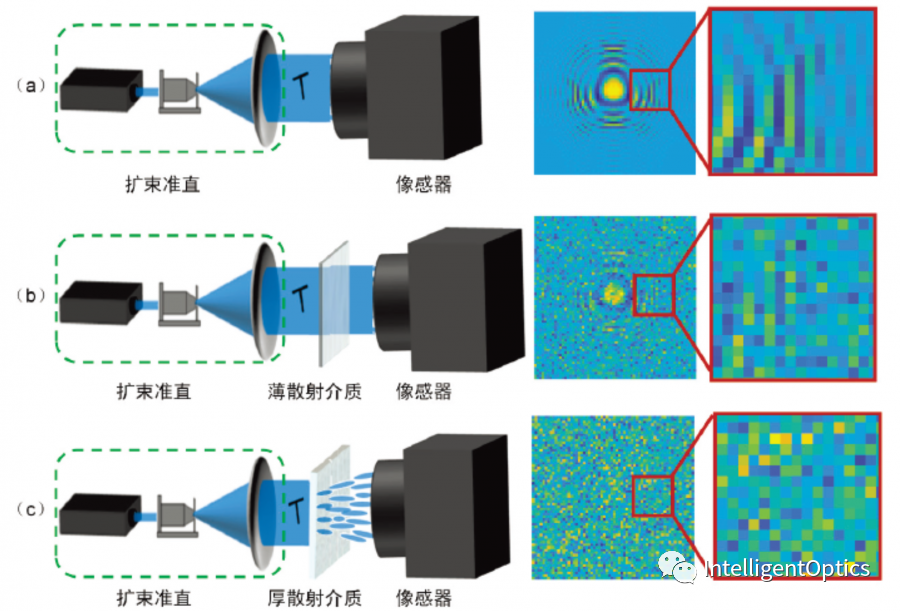图1 散射介质对无透镜全息成像的影响(文中用图由清华大学提供，下同)    论文对不同信噪比的全息编码图像采用压缩感知数字全息模型进行重建，结果表明无透镜压缩采样数字全息算法具有很强的抗噪声能力。由于全息编码的冗余性特点，物体上每一个像素的信息被编码到采样全息图的每一个像素上，非均匀介质产生的随机噪声不符合编码正向传输模型，在压缩感知重构过程中被有效的抑制，由此提高了成像系统的抗噪声能力。论文利用峰值信噪比(PSNR)和结构相似性(SSIM)两个指标定量分析了基于反传播算法和压缩感知数字全息算法的重建图像质量。研究表明压缩感知数字全息算法对于不同退化程度(-15dB~5dB)伽柏全息的重构结果都要好于传统的反传播算法。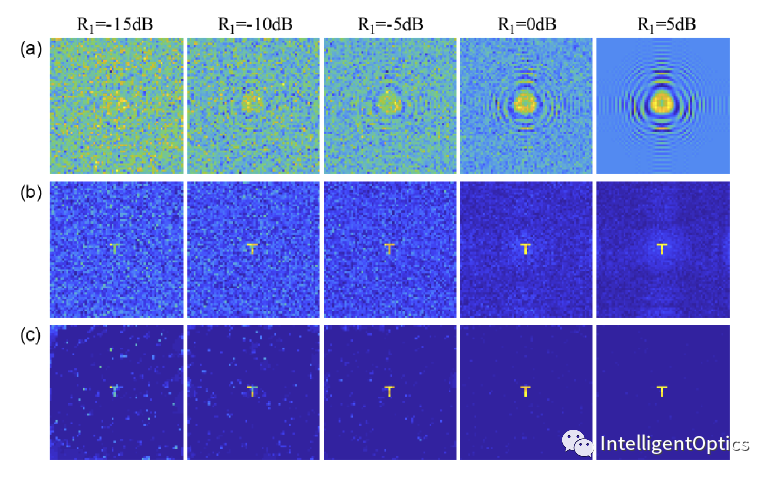图2 (a)在伽柏全息图中加入不同比例的噪声；(b)反传播算法重建结果；(c)压缩数字全息算法重建结果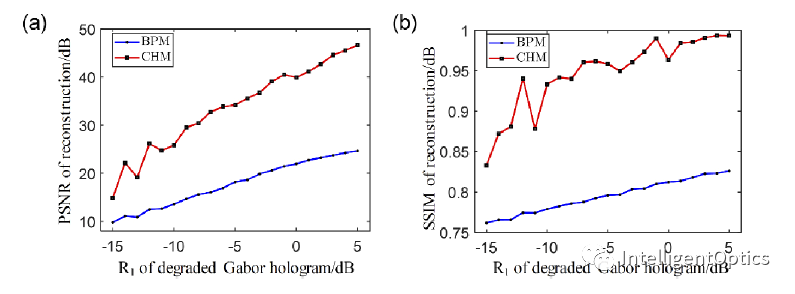图3 反传播算法和压缩数字全息算法重建结果PSNR(a)和SSIM(b)随伽柏全息图的SNR的变化    论文在实验中测量了两种不同类型的散射介质(随机相位板和磨砂玻璃DG10-220, Thorlabs)的光学深度，分别为1.42和6.38。针对透过随机相位板的弱散射介质成像，提出一次曝光采集的压缩数字全息重建方法，对实验采集的一幅全息图进行直接重建，相比于传统反传播算法重建结果的对比度为0.21，压缩数字全息成像重建结果的对比度明显优于传统算法，对比度可达0.48；对于透过磨砂玻璃的强散射介质成像，提出了多次曝光平均的压缩数字全息重建方法，通过采集多幅动态扰动全息图并做平均处理，压缩数字全息算法重建结果的对比度可达0.44。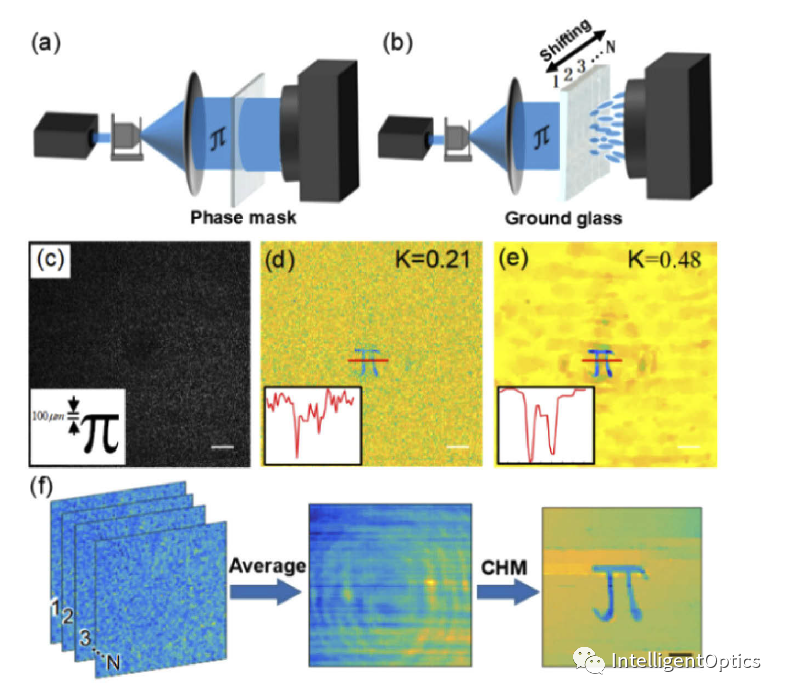图4 通过随机相位板(a)和磨砂玻璃(b)的成像系统，(c)透过弱散射介质采集到的伽柏全息图;(c)反传播算法的重建结果；(d)压缩数字全息算法的重建结果; (f)实验(b)的数据处理过程。    总结而言，该研究实现了透过非均匀散射介质的高质量重构，实验和仿真结果表明无透镜压缩感知数字全息算法具有很强的抗噪声能力，相比于传统反传播算法的重建结果有明显提升。该研究工作可广泛用基于弹道光子的非均匀介质成像，例如，可用于克服微流控芯片介质不均匀性和流体动态流动引起的全息图退化，实现高分辨率三维微流道流体成像。更多技术细节和代码详见Hua
Zhang, Songwen Liu, Liangcai Cao, and David J. Brady, "Noise
suppression for ballistic-photons based on compressive in-line
holographic imaging through an inhomogeneous medium," OSA Opt. Express
(2020).https://github.com/THUHoloLab/Imaging_through_inhomogeneous_medium回顾与预告上期回顾：显示专题(短评)| 基于重叠-相加全息立体图实现近眼显示 (ACM TOG) (欢迎点击查阅)下期预告：我们将不定期持续推荐学术领域具有代表性的计算显示和计算成像研究工作，同时穿插一些新型光学设计和VR/AR光机实现科普等的资讯分享，欢迎订阅关注，欢迎来稿交流。p.s. 本公众号IntelligentOpticsSharing (I.O.S.)运营背后以该领域的研究学者为主，非盈利非广告，希望能够结交共同兴趣方向的读者们，建立光学和计算机交叉学科领域内一个资讯分享交流的平台，还望大家多多海涵支持，欢迎拍砖。end展开全文• 针对现有光栅光谱解调方式所需数据量较大不利于数据传输及处理的现状，应用压缩感知算法通过少量光谱数据采集实现高精度光栅光谱的重构。选取可调谐法布里珀罗(F-P)滤波器解调方式（TFPDA）作为参照并以布拉格光栅...
• 压缩感知算法和变分模态分解相结合,应用于煤矿瓦斯数据的处理。考虑到现有的压缩感知算法在对瓦斯处理的过程中存在着重构精度低,重构过程复杂和需要较多的样本观测值等问题,因此提出一种基于VMD和自适应观测矩阵的...
• ## 压缩感知算法原理

千次阅读 2016-10-09 20:20:43
本文为笔者在学习压缩感知算法之后进行的整理
1. 本文中使用的符号一览

传统正交变换中：

符号
维度
描述

x$x$
RN×1$R^{N\times 1}$
原始信号

y$y$
RN×1$R^{N\times 1}$
压缩后的信号，不稀疏

y^$\hat{y}$
RN×1$R^{N\times 1}$
由y$y$中K$K$个分量放在对应位置构成，其余位置为0，是K$K$-稀疏的

压缩感知算法中：

符号
维度
描述

x$x$
RN×1$R^{N\times 1}$
原始信号

y$y$
RM×1$R^{M\times 1}$
压缩感知的观测向量，是x$x$的M$M$个线性度量

s$s$
RN×1$R^{N\times 1}$
信号x$x$在Ψ$\Psi$域的稀疏表示，是K$K$-稀疏的

Ψ$\Psi$
CN×N$C^{N\times N}$
稀疏矩阵，为正交变换矩阵

ΨH$\Psi^\mathrm{H}$
CN×N$C^{N\times N}$
正交变换矩阵Ψ$\Psi$的转置矩阵

Φ$\Phi$
RM×N$R^{M\times N}$
称为测量矩阵，有M≪N$M\ll N$

T$T$
RM×N$R^{M\times N}$
T=ΦΨH$T=\Phi\Psi^\mathrm{H}$，称为传感矩阵

δ$\delta$
1×1$1\times 1$
一个较小的正的常数

2. 压缩感知算法目的

压缩感知算法的目的是采集很少一部分数据，并希望从中“解压缩”出大量信息，达到恢复原信号的效果

3. 采集策略

a. 少量的采集到的数据包含了原信号的全局信息
b. 存在一种算法能够从这些少量的数据中还原出原先的信息来

4. 传统解决思路——正交变换

4.1 步骤

对于原始信号x∈RN×1$x\in R^{N\times 1}$，可以通过正交变换来进行压缩，正变换：y=Ψx$y=\Psi x$，反变换x=ΨHy$x=\Psi^\mathrm{H}y$。
这里Ψ$\Psi$是正交矩阵，有ΨΨH=ΨHΨ=I$\Psi\Psi^\mathrm{H}=\Psi^\mathrm{H}\Psi=I$，亦即ΨH=Ψ−1$\Psi^\mathrm{H}=\Psi^\mathrm{-1}$，Ψ∈CN×N$\Psi\in C^{N\times N}$，I$I$是单位矩阵。
对于y∈CN×1$y\in C^{N\times 1}$，能量较x$x$集中，本质上去除了x$x$中的相关性。因此，我们只保留K$K$个较大分量，而把其他N−K$N-K$个置为零，通过反变换，我们能够近乎完美的重建原始信号 ，具有这样性质的信号被称为K$K$“稀疏”的。

4.2 编码解码策略

编码：构造Ψ$\Psi$，做正变换y=Ψx$y=\Psi x$，保留y$y$中最重要的K$K$个分量和其对应位置。
解码：把K$K$个分量放回到对应位置，其他位置填上0，构造ΨH$\Psi^\mathrm{H}$，反变换x^=ΨHy^$\hat{x}=\Psi^\mathrm{H} \hat{y}$。

4.3 压缩误差分析

显然，我们希望∥x−x^∥2=∥y−y^∥2≤δ$\|x-\hat{x}\|_2=\|y-\hat{y}\|_2\leq \delta$，δ$\delta$是一个小的常数。
但更有效的是利用相对误差 ∥y−y^∥2∥y∥2≤δ$\frac{\|y-\hat{y}\|_2}{\|y\|_2}\leq\delta$。

5. 新的思路——压缩传感

5.1 压缩传感思想：

对于信号，x∈RN×1$x\in R^{N\times 1}$，我们可以找到它的M$M$个线性测量（Linear Measurement，意即降维后的信号为M$M$维），y=Φx，Φ∈RM×N$y=\Phi x，\Phi\in R^{M\times N}$。

Φ$\Phi$中的每一行看作一个传感器，它与信号相乘，拾取了信号的一部分信息。拥有了这M$M$个测量（即y$y$）和Φ$\Phi$，我们就可以近乎完美的重构原始信号了。

那么已知y$y$和Φ$\Phi$如何重构信号？这是一个最优化问题，根据以下式子:

目标函数min∥s∥0$min\|s\|_0$，且满足等式约束  ΦΨHs=y$\Phi\Psi^\mathrm{H}s=y$或者可以写成min∥y−ΦΨHs∥2+λ∥s∥0$min\|y-\Phi\Psi^\mathrm{H}s\|_2+\lambda\|s\|_0$

可以求得s$s$。
因为ΦΨHs=Φx^=y$\Phi\Psi^\mathrm{H}s=\Phi\hat{x}=y$，所以x^=ΨHs$\hat{x}=\Psi^\mathrm{H}s$，这里就是传统方法正交变换中用s$s$恢复x$x$的方法，这里的s$s$是K-sparse的。

5.2 根据以上分析，可以得出编码解码策略

编码：针对原始信号x$x$构造Φ$\Phi$，生成测量y=Φx$y=\Phi x$，保留y$y$。
解码：构造同样的Φ$\Phi$，构造任一种正交变换ΨH$\Psi^\mathrm{H}$，根据y$y$重构x$x$。

到这里，我们已经知道通过y$y$恢复x$x$的大致步骤，那么具体怎样操作呢？有这样三个关键问题：
(1) 观测矩阵Φ$\Phi$的选择需要满足什么性质？
(2) 如何最优化本文4.1中 min∥y−ΦΨHs∥2+λ∥s∥0$min\|y-\Phi\Psi^\mathrm{H}s\|_2+\lambda\|s\|_0$ 这个函数以求出s$s$呢？
下文将针对上述两个问题进行分析

5.3 观测矩阵Φ$\Phi$需要满足的性质

1. 随机性。Φ$\Phi$可以是高斯分布的白噪声矩阵，或伯努利分布的±1$\pm1$矩阵。
2. 使线性测量有稳定的能量性质，即满足1−δ≤∥ΦΨHs∥2∥s∥2≤1+δ$1-\delta\leq\frac{\|\Phi\Psi^\mathrm{H}s\|_2}{\|s\|_2}\leq1+\delta$，也就是它要保持K$K$个重要分量的长度。
3. 实际运用中，对Φ$\Phi$的维度有要求，y$y$的长度一般是重要分量长度的4倍，才能近乎完美重构。即M≈4K$M\approx4K$或者M≥Klog2(NK)$M\geq Klog_2(\frac{N}{K})$。

5.4 如何解决4.1中提出的优化问题

对于min∥y−ΦΨHs∥2+λ∥s∥0$min\|y-\Phi\Psi^\mathrm{H}s\|_2+\lambda\|s\|_0$ 的优化，其中的0-范数优化问题是NP难问题，因此我们必须要换成1-范数进行优化，而1-范数优化是一个凸优化问题。

5.4.1 为什么要将0-范数转换成1-范数而非2-范数进行优化呢？

我们把问题放到二维空间来看：
在二维空间里，假设s$s$有两个分量(s1,s2)$(s_1,s_2)$，则假设y$y$为1维向量，也就是一个数。
假设我们使用2-范数那么根据文章思路，令T=ΦΨH$T=\Phi\Psi^\mathrm{H}$，则有y=Ts$y=Ts$。
那么问题就变成了在满足等式约束y=Ts$y=Ts$的情况下，求∥s∥2$\|s\|_2$的最小值。
y=Ts$y=Ts$是二维空间中的一个超平面，为了简化，在2-D问题中看作一条直线；而∥s∥22=s21+s22$\|s\|_2^2=s_1^2+s_2^2$，即为半径为∥s∥2$\|s\|_2$的圆，要求该圆半径的最小值。直线和圆的关系在二维空间中如图所示：我们将圆的半径逐渐增大，直到与y=Ts$y=Ts$相切时，有∥s∥22$\|s\|_2^2$最小，此时圆与直线的交点坐标即为s$s$的两个分量值，此时的s$s$几乎不可能是稀疏的。
因此我们考虑y^$\hat{y}$的1-范数，那么问题变成了在满足y=Ts$y=Ts$的情况下，求∥s∥1$\|s\|_1$的最小值。∥s∥1=|s1|+|s2|$\|s\|_1=|s_1|+|s_2|$，该函数在二维空间中的图如下所示：我们将菱形边长从原点开始逐渐增大，直到与直线y=Ts$y=Ts$相切位置，菱形与直线的交点即为s$s$的两个分量值，此时有很大可能性该交点在坐标轴上，因此所求s$s$很可能是稀疏的。

5.4.2 对于1-范数的优化问题，如何进行求解？——正交匹配追踪法

对于y=Ts$y=Ts$,由于s$s$是K$K$稀疏的，我们要找其K$K$个分量，这K$K$个分量系数的绝对值应该比其他N−K$N-K$个分量的系数大得多。
我们先假设K=1$K=1$，此时唯一非零元素sq$s_q$在s$s$中对应的位置在q$q$。则Ts$Ts$就是恢复矩阵T$T$的第q$q$列Tq$T_q$与s$s$中的非零元素sq$s_q$的乘积，即Tqsq=yq$T_qs_q=y_q$，且∥y−yq∥2∥y∥2<δ$\frac{\|y-y_q\|_2}{\|y\|_2}<\delta$。换句话说，T$T$的第q$q$列与y$y$的相似程度最高，即|<Tq,y>|=|THqy|>>|THry|=|<Tr,y>|,r≠q$||=|T_q^\mathrm{H}y|>>|T_r^\mathrm{H}y|=||, r\neq q$。所以，我们只要计算恢复矩阵T$T$的所有列与y$y$的内积，找到内积绝对值最大的那列就行了，该列对应的位置就是q$q$。根据最小二乘法，sq=(THqTq)−1THqy$s_q=(T_q^\mathrm{H}T_q)^{-1}T_q^\mathrm{H}y$，就是使∥y−Tqsq∥2$\|y-T_qs_q\|_2$最小的那个sq$s_q$。此时余量rn=y−<Tq,y><Tq,Tq>Tq$r_n=y-\frac{}{}T_q$，始终同Tq$T_q$正交，很像施密特正交化方法。
当K=2$K=2$，则s$s$有两个非零元素sq1$s_{q1}$和sq2$s_{q2}$，其在s$s$中的位置分别为q1$q1$和q2$q2$。则T$T$的第q1$q1$和q2$q2$列与y$y$的相似程度最高。我们要找到使∥y−(Tq2,Tq1)(sq2sq1)∥2$\|y-(T_{q2},T_{q1})\begin{pmatrix} s_{q2}\\ s_{q1} \end{pmatrix}\|_2$最小的那个(sq2sq1)$\begin{pmatrix} s_{q2}\\ s_{q1} \end{pmatrix}$。这里Tq1$T_{q1}$是我们第一次找到的那一列，Tq2$T_{q2}$是我们要重新找的那一列。此时我们找到两个在变换域最关键的元素和其在s$s$中对应的位置了。令Tq=(Tq2,Tq1)$T_q=(T_{q2},T_{q1})$，余量rn$r_n$又一次被写为rn=y−<Tq,y><Tq,Tq>Tq$r_n=y-\frac{}{}T_q$。
当K>1$K>1$时，类似上面的步骤，迭代找到变换域中K$K$个最重要的分量。正交匹配的迭代次数m≥K$m\geq K$，实际上操作只要满足∥rn∥2∥y∥2<δ$\frac{\|r_n\|_2}{\|y\|_2}<\delta$，迭代就可以中止了。

主要参考：
1. http://blog.csdn.net/abcjennifer/article/details/7748833
2. 《“压缩传感”引论》.沙威.香港大学
展开全文图像处理
• 现有的自适应多尺度分块压缩感知算法忽略了高频信息在重建中的作用, 导致图像的边缘轮廓得不到充分重建; 并且在压缩分块过程中采用固定分块大小, 没有充分利用图像自身的稀疏性。针对上述不足, 提出一种多尺度分块的...
•研究论文
• 基于分块的压缩感知算法适用于图像信号的处理，通过平滑迭代阈值投影法可以快速重构图像，但存在低采样率下重构图像质量较差的缺点。基于全变差分的分块压缩感知算法，在一定程度上能提升重构效果，但降低了运算速度...
• 基于自适应压缩感知算法的光谱反射率重建关键技术研究研究论文
• 压缩感知算法（MATLAB编写，简单易懂，有注释）
• 里面包含CS去噪算法体系、去噪体系优缺点、视频去噪体系、重构算法总结四个文件，是本人读研三年的总结，适合压缩感知方向的初学者...